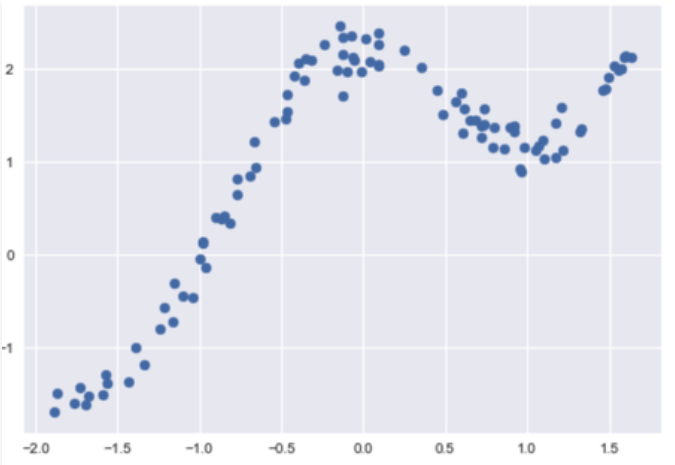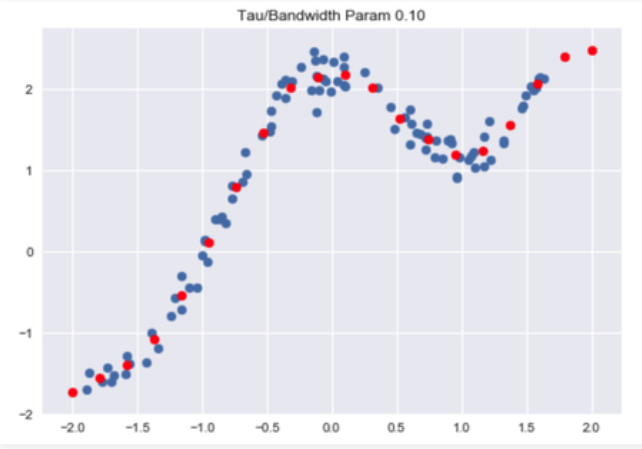# Implementation of Locally Weighted Linear Regression

LOESS or LOWESS are non-parametric regression methods that combine multiple regression models in a k-nearest-neighbor-based meta-model. LOESS combines much of the simplicity of linear least squares regression with the flexibility of nonlinear regression. It does this by fitting simple models to localized subsets of the data to build up a function that describes the variation in the data, point by point.

• This algorithm is used for making predictions when there exists a non-linear relationship between the features.
• Locally weighted linear regression is a supervised learning algorithm.
• It a non-parametric algorithm.
• doneThere exists No training phase. All the work is done during the testing phase/while making predictions.

Suppose we want to evaluate the hypothesis function h at a certan query point x. For linear regression we would do the following:For locally weighted linear regression we will instead do the following:where w(i) is a is a non-negative “weight” associated with training point x(i). A higher “preference” is given to the points in the training set lying in the vicinity of x than the points lying far away from x. so For x(i) lying closer to the query point x, the value of w(i) is large, while for x(i) lying far away from x the value of w(i) is small.
w(i) can be chosen as –Directly using closed Form solution to find parameters-Code: Importing Libraries :

 import numpy as np  import matplotlib.pyplot as plt  import pandas as pd     plt.style.use("seaborn")

 # Loading CSV files from local storage  dfx = pd.read_csv('weightedX_LOWES.csv')  dfy = pd.read_csv('weightedY_LOWES.csv')  # Getting data from DataFrame Object and storing in numpy n-dim arrays  X = dfx.values  Y = dfy.values

Output:Code: Function to calculate weight matrix :

 # function to calculate W weight diagnal Matric used in calculation of predictions  def get_WeightMatrix_for_LOWES(query_point, Training_examples, Bandwidth):    # M is the No of training examples    M = Training_examples.shape    # Initialising W with identity matrix    W = np.mat(np.eye(M))    # calculating weights for query points    for i in range(M):      xi = Training_examples[i]      denominator = (-2 * Bandwidth * Bandwidth)      W[i, i] = np.exp(np.dot((xi-query_point), (xi-query_point).T)/denominator)      return W

Code: Making Predictions:

 # function to make predictions  def predict(training_examples, Y, query_x, Bandwidth):    M = Training_examples.shape    all_ones = np.ones((M, 1))    X_ = np.hstack((training_examples, all_ones))    qx = np.mat([query_x, 1])    W = get_WeightMatrix_for_LOWES(qx, X_, Bandwidth)    # calculating parameter theta    theta = np.linalg.pinv(X_.T*(W * X_))*(X_.T*(W * Y))    # calculating predictions    pred = np.dot(qx, theta)    return theta, pred

Code: Visualise Predictions :

 # visualise predicted values with respect  # to original target values     Bandwidth = 0.1 X_test = np.linspace(-2, 2, 20)  Y_test = []  for query in X_test:    theta, pred = predict(X, Y, query, Bandwidth)    Y_test.append(pred)  horizontal_axis = np.array(X)  vertical_axis = np.array(Y)  plt.title("Tau / Bandwidth Param %.2f"% Bandwidth)  plt.scatter(horizontal_axis, vertical_axis)  Y_test = np.array(Y_test)  plt.scatter(X_test, Y_test, color ='red')  plt.show()Attention geek! Strengthen your foundations with the Python Programming Foundation Course and learn the basics.

To begin with, your interview preparations Enhance your Data Structures concepts with the Python DS Course.

My Personal Notes arrow_drop_upCheck out this Author's contributed articles.

If you like GeeksforGeeks and would like to contribute, you can also write an article using contribute.geeksforgeeks.org or mail your article to contribute@geeksforgeeks.org. See your article appearing on the GeeksforGeeks main page and help other Geeks.

Please Improve this article if you find anything incorrect by clicking on the "Improve Article" button below.